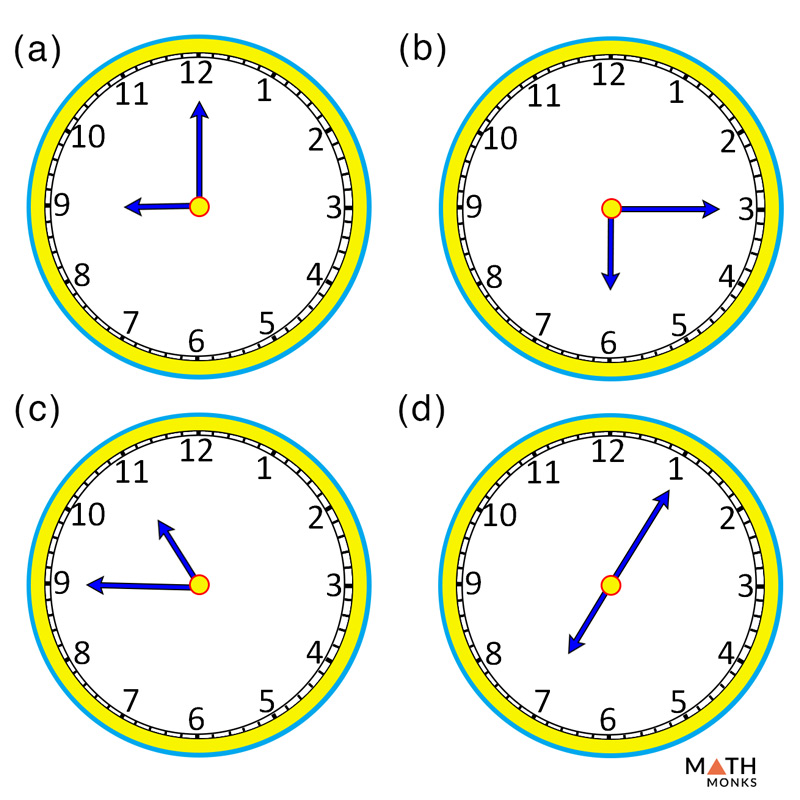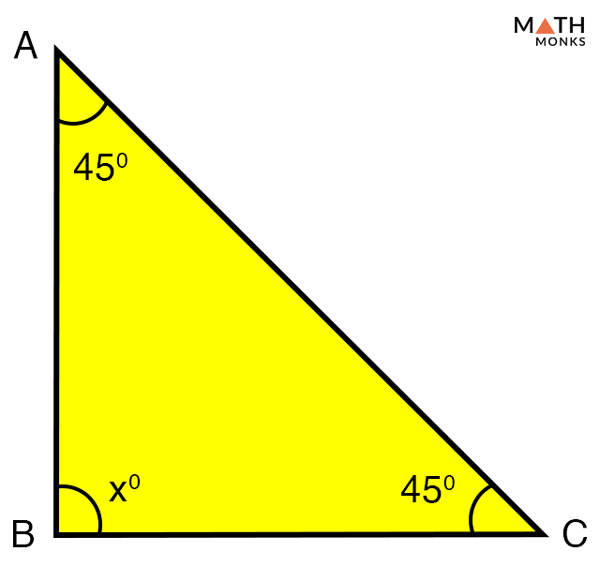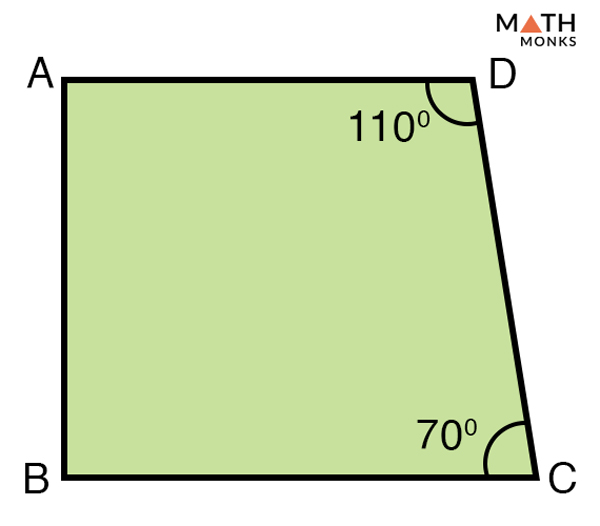# Right Angle

## What is a Right Angle

A right angle, also known as a 90-degree angle, is defined as an angle that measures exactly 90°. It is formed when two straight lines that are perpendicular to each other intersect at a point. A right angle is represented by the symbol ∟.

A few more right angles are shown below:

## How to Make a Right Angle

A 90-degree angle can be made by using only a scale and a compass. Follow the steps as shown below, to draw a 90-degree angle. Note that no protractor is used in any step of the process.

Figure 3 – (Heading: How to Make a 90 Degree Angle Using a Compass, Filename – How to Make a 90 Degree Angle)

## Right Angles in Real Life

• Geometric shapes such as square and rectangle have four right angles, a right trapezoid has two right angles, whereas a right angle triangle has one of its angle that is a right angle
• The two diagonals of some quadrilaterals such as a square, rhombus, or a kite intersect each other to form a right angle
• The angle formed by the hands of a clock showing 3’o clock and 9’ o clock
• Corners of square or rectangle-shaped swimming pools
• Corners of a paper, textbook, newspaper, and magazines
• Corners of a chessboard, table tennis board, ludo board, and billiard table
• Corners of a laptop and TV screen
• Corners of doors, windows, mirrors, and dinner table
• Corners of rooms in our homes, school, and offices
• The angle formed when a person is walking in relation to the ground
• Corners of each step of a staircase\Which of the following options given in the clock timing represents a right angle?

Solution:

As we know,
Right angles are angles that measure 90°
Hence, options (a) and (b) are right angles.Find the missing angle x in the given triangle and state whether it is a right angle triangle

Solution:

As we know,
The sum of the interior angles in a triangle is 180°,
Thus,
In △ABC,
∠ABC + ∠BCA + ∠CAB = 180°, here ∠CAB = 45°, ∠BCA = 45°
∠ABC + 45° + 45° = 180°
∠ABC = 180° – (45° + 45°)
∠ABC = (x°) = 90°
Since ∠ABC is 90°, △ABC is a right angle triangleIdentify the right angles in the given quadrilateral ABCD. Given that ∠DAB and ∠ABC are equal.

Solution:

As we know,
Sum of angles in a quadrilateral = 360°
Thus,
∠ABC + ∠BCD + ∠CDA + ∠DAB = 360°, here, ∠BCD = 70°, ∠CDA = 110°
∠ABC + 70° + 110° + ∠DAB = 360°
∠ABC +∠DAB = 360° – (70° + 110°)
∠ABC +∠DAB = 180°
Now, since ∠ABC = ∠DAB,
2∠ABC = 180°
∠ABC = 90°
Also, ∠DAB = 90°
Thus, in the given trapezoid ∠ABC and ∠DAB are right angles. Also the quadrilateral ABCD is a right trapezoid.

## FAQs

Q1. Does a rhombus have right angles?

Ans. Yes, for example a rhombus with four right angles is a square.

Q2. Does a parallelogram have right angles?

Ans. Yes, a parallelogram can have right angles as found in a square or a rectangle.

Q3. Does a trapezoid have right angles?

Ans. Yes, right trapezoid have right angles in them.

Q4. How many right angles does a square have?

Ans. A square has four right angles.

Q5. Do all quadrilaterals have right angles?

Ans. No, all quadrilaterals for example a kite does not have right angles.

Q6. How many right angles does a pentagon have?

Ans. A pentagon can have a maximum of three right angles.

Q7. Can a triangle have two right angles?

Ans. No, a triangle cannot have two right angles because the sum of the three angles in a triangle is 180°. If there are two right angles the measure of the third angles will be 0°, which is impossible.

Q8. How many right angles does a rectangle have?

Ans. A rectangle has four right angles.